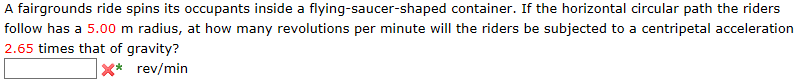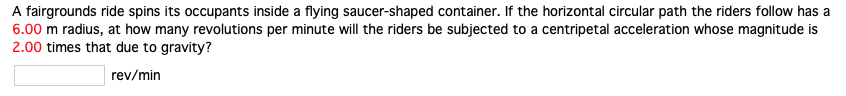Question
A centrifuge is a device in which small container of material is rotated at a high speed on a circular path. Such a device is a used in medical laboratories, for instance, to cause the more dense red blood cells to settle through the less dense blood serum and collect at the bottom of the container. Suppose the centripetal acceleration of the sample is 6.60x10^3 times as large as the acceleration due to gravity. How many revolutions per minute is the sample making, if it is located at a radius of 4.28 cm from the axis of rotation?

Calculate the angular velocity w (in radians per second) using the equation

R*w^2 = 6600 g = 64,700 m/s^2

Use R = 4.28*10^-2 m

w = 1230 rad/s

Convert that to rpm for the answer.

It's about 12,000 rpm.

#### Earn Coins

Coins can be redeemed for fabulous gifts.

Similar Homework Help Questions
• ### A centrifuge is a device in which a small container of material is rotated at a high speed on a circular path

A centrifuge is a device in which a small container of material is rotated at a high speed on a circular path. Such a device is used in medical laboratories, for instance, to cause the more dense red blood cells to settle through the less dense blood serum and collect at the bottom of the container. Suppose the centripetal acceleration of the sample is 4.47 x 103 times as large as the acceleration due to gravity. How many revolutions per...

• ### A centrifuge is a device in which a small container of material is rotated at a high speed on a circular path

A centrifuge is a device in which a small container of material is rotated at a high speed on a circular path. Such a device is used in medical laboratories, for instance, to cause the more dense red blood cells to settle through the less dense blood serum and collect at the bottom of the container. Suppose the centripetal acceleration of the sample is 5.65 103 times as large as the acceleration due to gravity. How many revolutions per minute...

• ### A centrifuge is a device in which a small container of material is rotated at a...

A centrifuge is a device in which a small container of material is rotated at a high speed on a circular path. Such a device is used in medical laboratories, for instance, to cause the more dense red blood cells to settle through the less dense blood serum and collect at the bottom of the container. Suppose the centripetal acceleration of the sample is 2.61 x 103 times as large as the acceleration due to gravity. How many revolutions per...

• ### Steve was subjected to accelerations in a horizontal "centrifuge". It is considered that such a device...

Steve was subjected to accelerations in a horizontal "centrifuge". It is considered that such a device has a radius of 5.0 m and can generate a centripetal acceleration equivalent to 7 times the average gravitational acceleration on Earth. a) For this maximum acceleration, what is the modulus of the astronaut's speed along the circular path? b) At how many revolutions per minute should the rotation occur to achieve such acceleration? c) What is the period of this rotation?

• ### A fairgrounds ride spins its occupants inside a flying-saucer-shaped container. If the horizontal circular path the...

A fairgrounds ride spins its occupants inside a flying-saucer-shaped container. If the horizontal circular path the riders follow has a 6.00 m radius, at how many revolutions per minute will the riders be subjected to a centripetal acceleration 2.95 times that of gravity?

• ### A fairgrounds ride spins its occupants inside a flying-saucer-shaped container. If the horizontal circular path the...

A fairgrounds ride spins its occupants inside a flying-saucer-shaped container. If the horizontal circular path the riders follow has a 7.00 m radius, at how many revolutions per minute will the riders be subjected to a centripetal acceleration 2.75 times that of gravity? rev/min

• ### A fairgrounds ride spins its occupants inside a flying-saucer-shaped container. If the horizontal circular path the...A fairgrounds ride spins its occupants inside a flying-saucer-shaped container. If the horizontal circular path the riders follow has a 5.00 m radius, at how many revolutions per minute will the riders be subjected to a centripetal acceleration 2.65 times that of gravity? x* rev/min

• ### A fairgrounds ride spins its occupants inside a flying-saucer-shaped container. If the horizontal circular path the...

A fairgrounds ride spins its occupants inside a flying-saucer-shaped container. If the horizontal circular path the riders follow has a 8.00 m radius, at how many revolutions per minute will the riders be subjected to a centripetal acceleration 2.85 times that of gravity? Answer in rev/min

• ### A fairgrounds ride spins its occupants inside a flying saucer-shaped container. If the horizontal circular path...

A fairgrounds ride spins its occupants inside a flying saucer-shaped container. If the horizontal circular path the riders follow has a 8.00 m radius, at how many revolutions per minute will the riders be subjected to a centripetal acceleration whose magnitude is 2.45 times that due to gravity?

• ### A fairgrounds ride spins its occupants inside a flying saucer-shaped container. If the horizontal circular path...A fairgrounds ride spins its occupants inside a flying saucer-shaped container. If the horizontal circular path the riders follow has a 6.00 m radius, at how many revolutions per minute will the riders be subjected to a centripetal acceleration whose magnitude is 2.00 times that due to gravity? rev/min